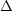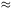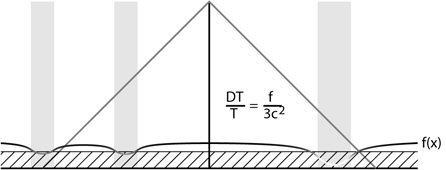### 1.1. INTRODUCTION

The first predictions of the anisotropy of the cosmic microwave background (CMB) were published shortly after the CMB was discovered by Penzias & Wilson (1965). Sachs & Wolfe (1967) calculated the anisotropies due to gravitational potential fluctuations produced by density perturbations (Figure 1.1). Because the density perturbations are given by the second derivative of the gravitational potential fluctuation in Poisson's equation, the Sachs-Wolfe effect dominates the temperature fluctuations at large scales or low spherical harmonic index. Sachs & Wolfe predictedT / T10-2 at large scales. This prediction, which failed by a factor of 103, is based on correct physics but incorrect input assumptions: prior to the discovery of the CMB no one knew how uniform the Universe was on large scales.Figure 1.1. Sachs & Wolfe (1967) predicted that density enhancements would be cold spots in the CMB, as shown in this conformal spacetime diagram.

Silk (1968) computed the density perturbations needed at the recombination epoch at z103 in order to produce galaxies, and predictedT / T3 × 10-4 on arcminute scales. Silk (1967) calculated the damping of waves that were partially optically thick during recombination. This process, known as "Silk damping," greatly reduces the CMB anisotropy for small angular scales.

Observations by Conklin (1969) and then Henry (1971) showed that there was a dipole anisotropy in the CMB corresponding to the motion of the Solar System with respect to the average velocity of the observable Universe. There is a discussion of the dipole observations and their interpretation in Peebles (1971) that is still valid today, except that what was then a "tentative" dipole is now known to better than 1% accuracy, after a string of improved measurements starting with Corey & Wilkinson (1976) and ending with the COBE DMR (Bennett et al. 1996).

Peebles & Yu (1970) calculated the baryonic oscillations resulting from interactions between photons and hydrogen in the early Universe, and also independently introduced the Harrison-Zel'dovich spectrum (Harrison 1970; Zel'dovich 1972). Pebbles & Yu predictedT / T1.5 × 10-4 on 1' scales andT / T1.7 × 10-3 on 7' scales.

Wilson & Silk (1981) further developed the theory of photon and matter interaction by scattering and gravity, and predictedT / T = 100 µK for a single subtracted experiment with a 7° throw and with a 7° beam like COBE. Of course, when COBE was launched in 1989 it actually observed a much smaller anisotropy.

These early predictions of a large anisotropy were greatly modified by the addition of dark matter to the recipe for the cosmos. Observational upper limits on small-scale anisotropies had reachedT / T < 4 × 10-5 on 1.'5 scales (Uson & Wilkinson 1982), which was considerably less than the predictions from universes with just baryons and photons. Peebles (1982) computed the anisotropy expected in a universe "dominated by massive, weakly interacting particles" - in other words cold dark matter (CDM), although this paper predated the use of "cold dark matter."

Bond & Efstathiou (1987) calculated the correlation function of the CMB anisotropy, C(), and also the angular power spectrum, C, in the CDM cosmology. This paper contains one of the first plots showing(+ 1)Cvs., with peaks originally called the "Doppler" peaks but more properly called "acoustic peaks." This paper solved the Boltzmann equation describing the evolution of the photon distribution functions. Several authors developed these "Boltzmann codes," but the calculation of the angular power spectrum up to highwas very slow. These codes described the conversion of inhomogeneity at the last-scattering surface into anisotropy on the observed sky by a set of differential equations evolving the coefficients of a Legendre polynomial expansion of the radiation intensity. Since the Universe is almost completely transparent after recombination, a ray-tracing approach is much more efficient. This great step in efficiency was implemented in the CMBFAST code by Seljak & Zaldarriaga (1996).

Hu & Dodelson (2002) give a recent review of CMB anisotropies, which includes a very good tutorial on the theory ofT / T.